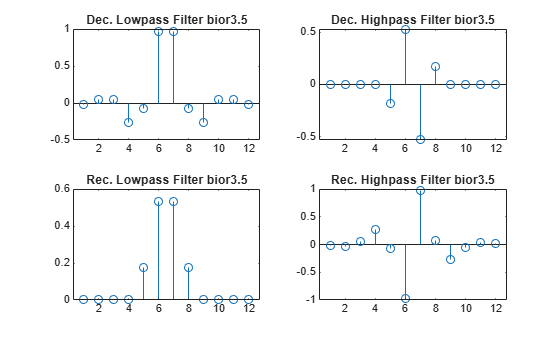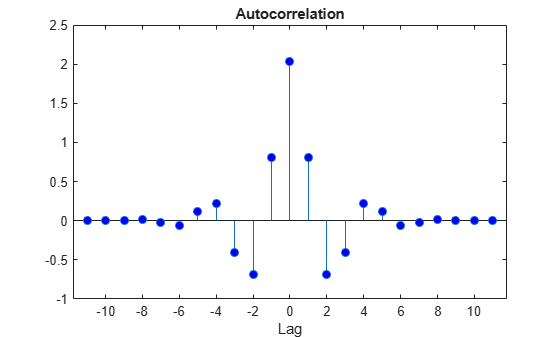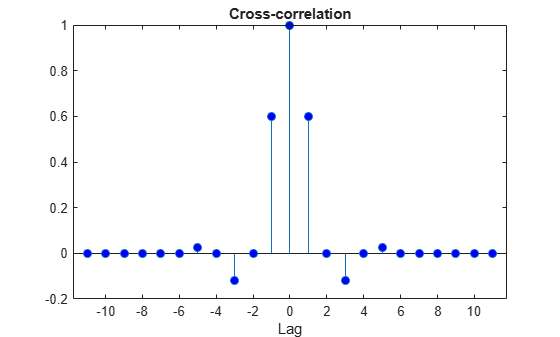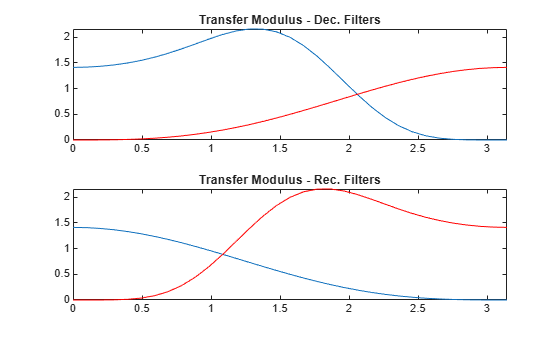# biorfilt

Biorthogonal wavelet filter set

## Syntax

``[LoD,HiD,LoR,HiR] = biorfilt(DF,RF)``
``[LoD1,HiD1,LoR1,HiR1,LoD2,HiD2,LoR2,HiR2] = biorfilt(DF,RF,'8')``

## Description

example

````[LoD,HiD,LoR,HiR] = biorfilt(DF,RF)` returns four filters associated with the biorthogonal wavelet specified by decomposition filter `DF` and reconstruction filter `RF`. These filters are `LoD` — Decomposition lowpass filter`HiD` — Decomposition highpass filter`LoR` — Reconstruction lowpass filter`HiR` — Reconstruction highpass filter ```
````[LoD1,HiD1,LoR1,HiR1,LoD2,HiD2,LoR2,HiR2] = biorfilt(DF,RF,'8')` returns eight filters, the first four associated with the decomposition wavelet, and the last four associated with the reconstruction wavelet.```

## Examples

collapse all

This example shows how to obtain the decomposition (analysis) and reconstruction (synthesis) filters for the `'bior3.5'` wavelet.

Obtain the two scaling and wavelet filters associated with the `'bior3.5'` wavelet.

```wv = 'bior3.5'; [Rf,Df] = biorwavf(wv); [LoD,HiD,LoR,HiR] = biorfilt(Df,Rf);```

Plot the filter impulse responses.

```subplot(2,2,1) stem(LoD) title(['Dec. Lowpass Filter ',wv]) subplot(2,2,2) stem(HiD) title(['Dec. Highpass Filter ',wv]) subplot(2,2,3) stem(LoR) title(['Rec. Lowpass Filter ',wv]) subplot(2,2,4) stem(HiR) title(['Rec. Highpass Filter ',wv])```Demonstrate that autocorrelations at even lags are only zero for dual pairs of filters. Examine the autocorrelation sequence for the lowpass decomposition filter.

```npad = 2*length(LoD)-1; LoDxcr = fftshift(ifft(abs(fft(LoD,npad)).^2)); lags = -floor(npad/2):floor(npad/2); figure stem(lags,LoDxcr,'markerfacecolor',[0 0 1]) set(gca,'xtick',-10:2:10) title('Autocorrelation') xlabel('Lag')```Examine the cross-correlation sequence for the lowpass decomposition and synthesis filters. Compare the result with the preceding figure. At even lags, the cross-correlation is zero.

```npad = 2*length(LoD)-1; xcr = fftshift(ifft(fft(LoD,npad).*conj(fft(LoR,npad)))); lags = -floor(npad/2):floor(npad/2); stem(lags,xcr,'markerfacecolor',[0 0 1]) set(gca,'xtick',-10:2:10) title('Cross-correlation') xlabel('Lag')```Compare the transfer functions of the analysis and synthesis scaling and wavelet filters.

```dftLoD = fft(LoD,64); dftLoD = dftLoD(1:length(dftLoD)/2+1); dftHiD= fft(HiD,64); dftHiD = dftHiD(1:length(dftHiD)/2+1); dftLoR = fft(LoR,64); dftLoR = dftLoR(1:length(dftLoR)/2+1); dftHiR = fft(HiR,64); dftHiR = dftHiR(1:length(dftHiR)/2+1); df = (2*pi)/64; freqvec = 0:df:pi; subplot(2,1,1) plot(freqvec,abs(dftLoD),freqvec,abs(dftHiD),'r') axis tight title('Transfer Modulus - Dec. Filters') subplot(2,1,2) plot(freqvec,abs(dftLoR),freqvec,abs(dftHiR),'r') axis tight title('Transfer Modulus - Rec. Filters')```## Input Arguments

collapse all

Decomposition scaling filter associated with a biorthogonal wavelet, specified as a vector.

Data Types: `double`

Reconstruction scaling filter associated with a biorthogonal wavelet, specified as a vector.

Data Types: `double`

## Output Arguments

collapse all

Wavelet decomposition filters, returned as a pair of even-length real-valued vectors. `LoD` is the lowpass decomposition filter, and `HiD` is the highpass decomposition filter.

Wavelet reconstruction filters, returned as a pair of even-length real-valued vectors. `LoR` is the lowpass reconstruction filter, and `HiR` is the highpass reconstruction filter.

Filters associated with the decomposition (analysis) wavelet, returned as even-length real-valued vectors.

• `LoD1` — Decomposition lowpass filter

• `HiD1` — Decomposition highpass filter

• `LoR1` — Reconstruction lowpass filter

• `HiR1` — Reconstruction highpass filter

Filters associated with the reconstruction (synthesis) wavelet, returned as even-length real-valued vectors.

• `LoD2` — Decomposition lowpass filter

• `HiD2` — Decomposition highpass filter

• `LoR2` — Reconstruction lowpass filter

• `HiR2` — Reconstruction highpass filter

collapse all

### Biorthogonal Filters

It is well known in the subband filtering community that if the same FIR filters are used for reconstruction and decomposition, then symmetry and exact reconstruction are incompatible (except with the Haar wavelet). Therefore, with biorthogonal filters, two wavelets are introduced instead of just one.

One wavelet, $\stackrel{˜}{\psi }$, is used in the analysis, and the coefficients of a signal s are

`${\stackrel{˜}{c}}_{j,k}=\int s\left(x\right){\stackrel{˜}{\psi }}_{j,k}\left(x\right)dx$`

The other wavelet, ψ, is used in the synthesis:

`$s=\sum _{j,k}{\stackrel{˜}{c}}_{j,k}{\psi }_{j,k}$`

Furthermore, the two wavelets are related by duality in the following sense:
$\int {\stackrel{˜}{\psi }}_{j,k}\left(x\right){\psi }_{{j}^{\prime },{k}^{\prime }}\left(x\right)dx=0$ as soon as j ≠ j′ or k ≠ k′ and
$\int {\stackrel{˜}{\varphi }}_{0,k}\left(x\right){\varphi }_{0,{k}^{\prime }}\left(x\right)dx=0$ as soon as k ≠ k′.

It becomes apparent, as A. Cohen pointed out in his thesis (p. 110), that “the useful properties for analysis (e.g., oscillations, null moments) can be concentrated in the $\stackrel{˜}{\psi }$ function; whereas, the interesting properties for synthesis (regularity) are assigned to the ψ function. The separation of these two tasks proves very useful.”

$\stackrel{˜}{\psi }$ and ψ can have very different regularity properties, ψ being more regular than $\stackrel{˜}{\psi }$.

The $\stackrel{˜}{\psi }$, ψ, $\stackrel{˜}{\varphi }$ and ϕ functions are zero outside a segment.

 Cohen, Albert. "Ondelettes, analyses multirésolution et traitement numérique du signal," Ph. D. Thesis, University of Paris IX, DAUPHINE. 1992.

 Daubechies, Ingrid. Ten Lectures on Wavelets. CBMS-NSF Regional Conference Series in Applied Mathematics 61. Philadelphia, Pa: Society for Industrial and Applied Mathematics, 1992.